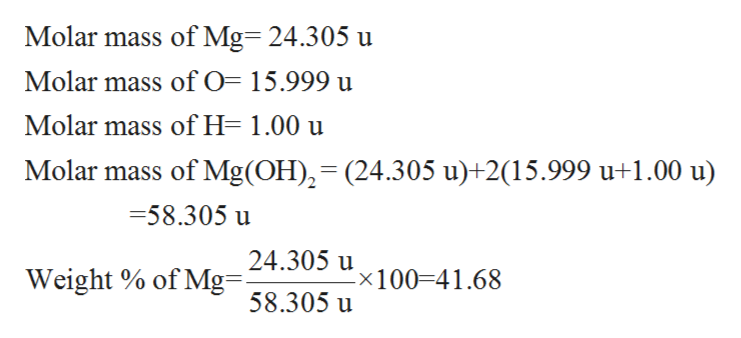# How do you find the weight percentage of Mg in Mg(OH)2, Mg3N2, and MgO?

Question
159 views

How do you find the weight percentage of Mg in Mg(OH)2, Mg3N2, and MgO?

check_circle

Step 1

Percent by mass or percent by weight refers to the total mass of the one component divided by the mass of the solution.

Step 2

Firstly, the mass of the given component is divided by the mass of one mole of the given compound and then the resultant fraction is multiplied by 100.

Therefore, the percent by weight can be find as follows:

Step 3

First compound is given to be Mg (OH)2. Its molar...help_outlineImage TranscriptioncloseMolar mass of Mg 24.305 u Molar mass of O= 15.999 u Molar mass of H- 1.00 u Molar mass of Mg(OH)2=(24.305 u)+2(15.999 u+1.00 u) =58.305 u 24.305 u Weight % of Mg 8.305 u x100 41.68 fullscreen

### Want to see the full answer?

See Solution

#### Want to see this answer and more?

Solutions are written by subject experts who are available 24/7. Questions are typically answered within 1 hour.*

See Solution
*Response times may vary by subject and question.
Tagged in

### Inorganic Chemistry